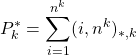# Volume 2, 1996, Number 2

Volume 2Number 1 ▷ Number 2 ▷ Number 3Number 4

The Goldbach problem. II (Continuation)
Original research paper. Pages 1–26
Aldo Peretti
Full paper (PDF, 628 Kb)

Necessary and sufficient conditions for simple A-bases
Original research paper. Pages 27–39
Calvin Long and Carl Swenson
Full paper (PDF, 390 Kb)

The unitary analogue of Pillai’s arithmetical functions. II
Original research paper. Pages 40–46
László Tóth
Full paper (PDF, 277 Kb) | Abstract

Let k be a positive integer and letdenote the greatest k-th power divisor of a which is a unitary divisor of b. We introduce the functionand obtain the arithmetical evaluation of it and an asymptotic formula for the summatory function of, which improves for k = 1 an earlier result of the author.

A modification of one Avanessov’s problem
Original research paper. Pages 47–48
Krassimir T. Atanassov
Full paper (PDF, 87 Kb)

Volume 2Number 1 ▷ Number 2 ▷ Number 3Number 4